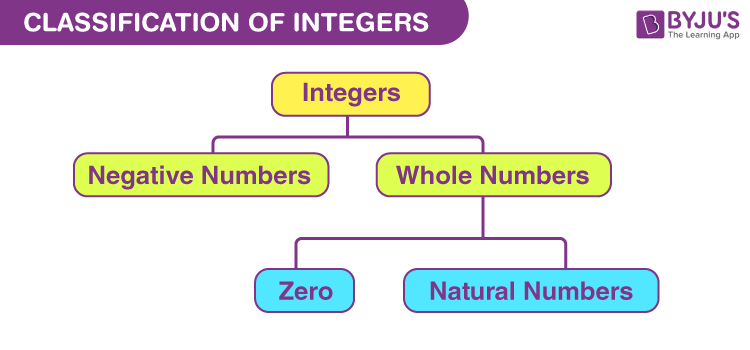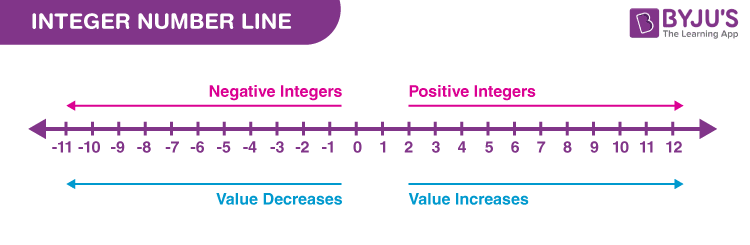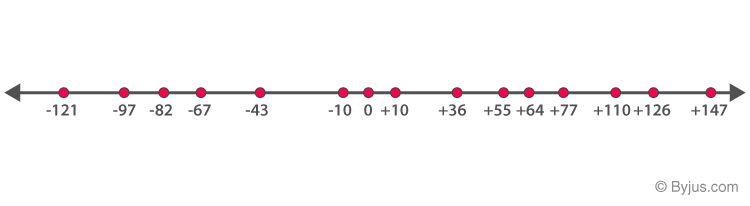# Integers

In Mathematics, integers are the collection of whole numbers and negative numbers. Similar to whole numbers, integers also does not include the fractional part. Thus, we can say, integers are numbers that can be positive, negative or zero, but cannot be a fraction. We can perform all the arithmetic operations, like addition, subtraction, multiplication and division, on integers. The examples of integers are, 1, 2, 5,8, -9, -12, etc. The symbol of integers is “Z“. Now, let us discuss the definition of integers, symbol, types, operations on integers, rules and properties associated to integers, how to represent integers on number line with many solved examples in detail.

## What are Integers?

The word integer originated from the Latin word “Integer” which means whole or intact. Integers is a special set of numbers comprising zero, positive numbers and negative numbers.

Examples of Integers: – 1, -12, 6, 15.

### Symbol

The integers are represented by the symbol ‘Z’.

Z= {……-8,-7,-6, -5, -4, -3, -2, -1, 0, 1, 2, 3, 4, 5, 6, 7, 8,……}

## Types of Integers

Integers come in three types:

• Zero (0)
• Positive Integers (Natural numbers)
• Negative Integers (Additive inverse of Natural Numbers)

### Zero

Zero is neither a positive nor a negative integer. It is a neutral number i.e. zero has no sign (+ or -).

### Positive Integers

The positive integers are the natural numbers or also called counting numbers. These integers are also sometimes denoted by Z+. The positive integers lie on the right side of 0 on a number line.

 Z+ → 1, 2, 3, 4, 5, 6, 7, 8, 9, 10, 11, 12, 13, 14, 15, 16, 17, 18, 19, 20, 21, 22, 23, 24, 25, 26, 27, 28, 29, 30,….

### Negative Integers

The negative integers are the negative of natural numbers. They are denoted by Z. The negative integers lie on the left side of 0 on a number line.

 Z– → -1, -2, -3, -4, -5, -6, -7, -8, -9, -10, -11, -12, -13, -14, -15, -16, -17, -18, -19, -20, -21, -22, -23, -24, -25, -26, -27, -28, -29, -30,…..## How to Represent Integers on Number Line?

As we have already discussed the three categories of integers, we can easily represent them on a number line based on positive integers, negative integers and zero.

Zero is the centre of integers on a number line. Positive integers lie on the right side of zero and negative integers lie on the left. See the below figure.## Rules of Integers

Rules defined for integers are:

• Sum of two positive integers is an integer
• Sum of two negative integers is an integer
• Product of two positive integers is an integer
• Product of two negative integers is an integer
• Sum of an integer and its inverse is equal to zero
• Product of an integer and its reciprocal is equal to 1

## Arithmetic Operations on Integers

The basic Maths operations performed on integers are:

• Subtraction of integers
• Multiplication of integers
• Division of integers

While adding the two integers with the same sign, add the absolute values, and write down the sum with the sign provided with the numbers.

For example,

(+4) + (+7) = +11

(-6) + (-4) = -10

While adding two integers with different signs, subtract the absolute values, and write down the difference with the sign of the number which has the largest absolute value.

For example,

(-4) + (+2) = -2

(+6) + (-4) = +2.

### Subtraction of Integers

While subtracting two integers, change the sign of the second number which is being subtracted, and follow the rules of addition.

For example,

(-7) – (+4) = (-7) + (-4) = -11

(+8) – (+3) = (+8) + (-3) = +5

### Multiplication of Integers

While multiplying two integer numbers, the rule is simple.

• If both the integers have the same sign, then the result is positive.
• If the integers have different signs, then the result is negative.

For example,

• (+2) x (+3) = +6
• (+3) x (-4) = – 12

Thus, we can summarise the multiplication of two integers with examples in the below table.

 Multiplication of Signs Resulting Sign Examples + × + + 3 × 4 = 12 + × – – 3 × -4 = -12 – × + – -3 × 4 = -12 – × – + -3 × -4 = 12

### Division of Integers

The rule for dividing integers is similar to multiplication.

• If both the integers have the same sign, then the result is positive.
• If the integers have different signs, then the result is negative.

Similarly

• (+6) ÷ (+2) = +3
• (-16) ÷ (+4) = -4
 Division of Signs Resulting sign Examples + ÷ + + 15 ÷ 3 = 5 + ÷ – – 15 ÷ -3 = -5 – ÷ + – -15 ÷ 3 = -5 – ÷ – + -15 ÷ -3 = 5

## Properties of Integers

The major Properties of Integers are:

1. Closure Property
2. Associative Property
3. Commutative Property
4. Distributive Property
6. Multiplicative Inverse Property
7. Identity Property

### Closure Property

According to the closure property of integers, when two integers are added or multiplied together, it results in an integer only. If a and b are integers, then:

• a + b = integer
• a x b = integer

Examples:

• 2 + 5 = 7 (is an integer)
• 2 x 5 = 10 (is an integer)

### Commutative Property

According to the commutative property of integers, if a and b are two integers, then:

• a + b = b + a
• a x b = b x a

Examples:

• 3 + 8 = 8 + 3 = 11
• 3 x 8 = 8 x 3 = 24

But for the commutative property is not applicable to subtraction and division of integers.

### Associative Property

As per the associative property , if a, b and c are integers, then:

• a+(b+c) = (a+b)+c
• ax(bxc) = (axb)xc

Examples:

• 2+(3+4) = (2+3)+4 = 9
• 2x(3×4) = (2×3)x4 = 24

Similar to commutativity, associativity is applicable for the addition and multiplication of integers only.

### Distributive property

According to the distributive property of integers, if a, b and c are integers, then:

a x (b + c) = a x b + a x c

Example: Prove that: 3 x (5 + 1) = 3 x 5 + 3 x 1

LHS = 3 x (5 + 1) = 3 x 6 = 18

RHS = 3 x 5 + 3 x 1 = 15 + 3 = 18

Since, LHS = RHS

Hence, proved.

If a is an integer, then as per the additive inverse property of integers,

a + (-a) = 0

Hence, -a is the additive inverse of integer a.

### Multiplicative inverse Property

If a is an integer, then as per the multiplicative inverse property of integers,

a x (1/a) = 1

Hence, 1/a is the multiplicative inverse of integer a.

### Identity Property of Integers

The identity elements of integers are:

• a+0 = a
• a x 1 = a

Example: -100,-12,-1, 0, 2, 1000, 989 etc…

As a set, it can be represented as follows:

Z= {……-8,-7,-6, -5, -4, -3, -2, -1, 0, 1, 2, 3, 4, 5, 6, 7, 8,……}

## Applications of Integers

Integers are not just numbers on paper; they have many real-life applications. The effect of positive and negative numbers in the real world is different. They are mainly used to symbolize two contradicting situations.

For example, when the temperature is above zero, positive numbers are used to denote temperature, whereas negative numbers indicate the temperature below zero. They help one to compare and measure two things like how big or small or more or fewer things are and hence can quantify things.

Some real-life situations where integers come into play are player’s scores in golf, football and hockey tournaments, the rating of movies or songs, in banks credits and debits are represented as positive and negative amounts respectively.

## Examples on Integers

Example 1:

Plot the following integers on the number line:

-121, -97, -82, -67, -43, -10, 0, 10, 36, 55, 64, 77, 110, 126, 147.

Solution:In the above number line, each number has been plotted with a red dot.

Example 2:

Solve the following:

• 5 + 3 = ?
• 5 + (-3) = ?
• (-5) + (-3) = ?
• (-5) x (-3) = ?

Solution:

• 5 + 3 = 8
• 5 + (-3) = 5 – 3 = 2
• (-5) + (-3) = -5 – 3 = -8
• (-5) x (-3) = 15

Example 3:

Solve the following product of integers:

• (+5) × (+10)
• (12) × (5)
• (- 5) × (7)
• 5 × (-4)

Solution:

• (+5) × (+10) = +50
• (12) × (5) = 60
• (- 5) × (7) = -35
• 5 × (-4) = -20

Example 4:

Solve the following division of integers:

• (-9) ÷ (-3)
• (-18) ÷ (3)
• (4000) ÷ (- 100)

Solution:

• (-9) ÷ (-3) = 3
• (-18) ÷ (3) = -6
• (4000) ÷ (- 100) = -40

## Practice Questions on Integers

1. Sum of two positive integers is a positive integer. True or False?
2. What is the sum of first five positive integers?
3. What is the product of first five positive odd integers?
4. Plot the integers from -10 to +10 on the number line.

To learn about the properties of integers and to solve problems on the topic, download BYJU’S – The Learning App from Google Play Store and watch interactive videos.

## Frequently Asked Questions on Integers

### What are integers?

Integers are the combination of zero, natural numbers and their additive inverse. It can be represented in a number line excluding the fractional part. It is denoted by Z.

### What is an integer formula?

An integer is a set of positive and negative numbers along with zero and does not have any formula.

### What are the examples of integers?

The examples of integers are 3, -5, 0, 99, -45, etc.

### Can integers be negative?

Yes, the integers can be negative. Additive inverse of natural numbers are negative integers, such as -1,-2,-3,-4,-5, and so on

### What are the types of integers?

Integers are of three types:
Zero, Positive integers and Negative integers

Quiz on Integers

1. Sanjana

Class 7 explain all chapters

The set of positive and negative integers together with 0 is called